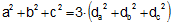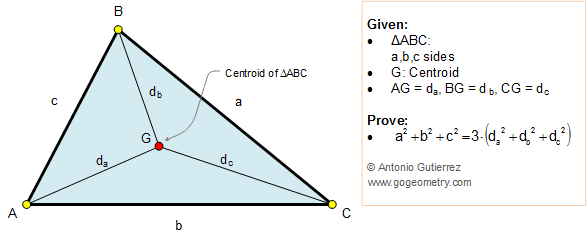Problem 253. Triangle, Centroid, Vertices, Distances, Squares The figure shows a triangle ABC and the centroid G (point of concurrency of the triangle's medians). If AB = c, BC = a, AC = b, AG = da, BG = db, and CG = dc, prove that. View or post a solution.Home | Sitemap | Geometry | Problems | 251-260 | View or post a solution